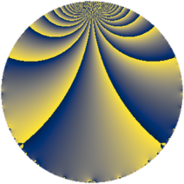# Properties

 Label 48.2.kLevel $48$ Weight $2$ Character orbit 48.k Rep. character $\chi_{48}(11,\cdot)$ Character field $\Q(\zeta_{4})$ Dimension $12$ Newform subspaces $1$ Sturm bound $16$ Trace bound $0$

# Related objects

## Defining parameters

 Level: $$N$$ $$=$$ $$48 = 2^{4} \cdot 3$$ Weight: $$k$$ $$=$$ $$2$$ Character orbit: $$[\chi]$$ $$=$$ 48.k (of order $$4$$ and degree $$2$$) Character conductor: $$\operatorname{cond}(\chi)$$ $$=$$ $$48$$ Character field: $$\Q(i)$$ Newform subspaces: $$1$$ Sturm bound: $$16$$ Trace bound: $$0$$

## Dimensions

The following table gives the dimensions of various subspaces of $$M_{2}(48, [\chi])$$.

Total New Old
Modular forms 20 20 0
Cusp forms 12 12 0
Eisenstein series 8 8 0

## Trace form

 $$12 q - 2 q^{3} - 4 q^{4} - 8 q^{6} - 8 q^{7} + O(q^{10})$$ $$12 q - 2 q^{3} - 4 q^{4} - 8 q^{6} - 8 q^{7} - 8 q^{12} - 4 q^{13} + 16 q^{16} + 4 q^{18} - 12 q^{19} - 8 q^{21} + 16 q^{22} + 24 q^{24} + 10 q^{27} - 8 q^{28} + 28 q^{30} - 4 q^{33} - 8 q^{34} + 20 q^{36} - 4 q^{37} + 20 q^{39} - 40 q^{40} - 24 q^{42} + 12 q^{43} - 12 q^{45} - 40 q^{46} - 48 q^{48} - 20 q^{49} + 24 q^{51} - 16 q^{52} - 52 q^{54} + 24 q^{55} + 32 q^{58} - 16 q^{60} + 12 q^{61} + 56 q^{64} + 28 q^{66} + 28 q^{67} + 4 q^{69} + 40 q^{70} + 40 q^{72} - 34 q^{75} + 56 q^{76} + 60 q^{78} - 4 q^{81} - 16 q^{82} + 16 q^{84} + 32 q^{85} - 60 q^{87} - 64 q^{88} - 16 q^{90} - 56 q^{91} + 28 q^{93} - 48 q^{94} - 56 q^{96} - 8 q^{97} - 52 q^{99} + O(q^{100})$$

## Decomposition of $$S_{2}^{\mathrm{new}}(48, [\chi])$$ into newform subspaces

Label Dim $A$ Field CM Traces $q$-expansion
$a_{2}$ $a_{3}$ $a_{5}$ $a_{7}$
48.2.k.a $12$ $0.383$ 12.0.$$\cdots$$.2 None $$0$$ $$-2$$ $$0$$ $$-8$$ $$q+\beta _{6}q^{2}-\beta _{10}q^{3}+(-\beta _{1}+\beta _{10}+\beta _{11})q^{4}+\cdots$$